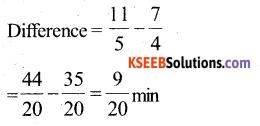# KSEEB Solutions for Class 6 Maths Chapter 7 Fractions Ex 7.6

Students can Download Chapter 7 Fractions Ex 7.6 Questions and Answers, Notes Pdf, KSEEB Solutions for Class 6 Maths helps you to revise the complete Karnataka State Board Syllabus and score more marks in your examinations.

## Karnataka State Syllabus Class 6 Maths Chapter 7 Fractions Ex 7.6

Question 1.
Solve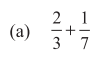Solution: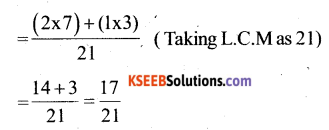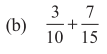Solution: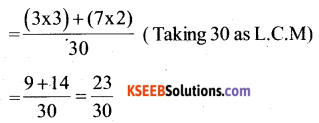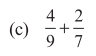Solution: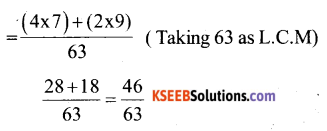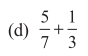Solution: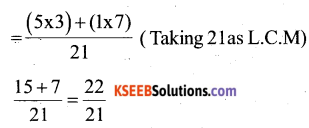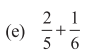Solution: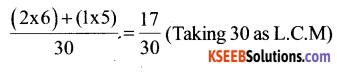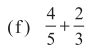Solution: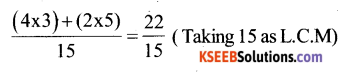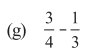Solution: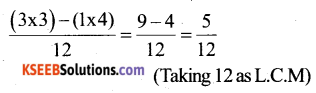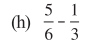Solution: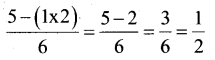Taking 6 as L.C.M.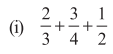Solution: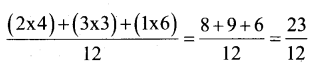(Taking 12 as L.C.M)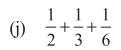Solution: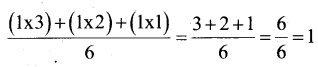(Taking 6 as L.C.M)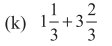Solution: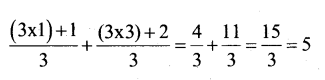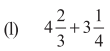Solution: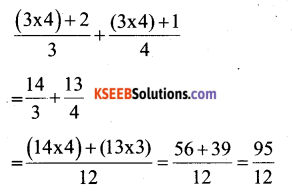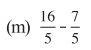Solution: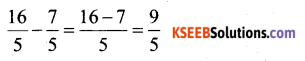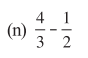Solution:Question 2.
Santa bought $$\frac{2}{5}$$ metre of ribbon and Lalita $$\frac{3}{4}$$ metre of ribbon. What is the total length of the ribbon they bought?
Solution:
Length of ribbon bought by Sarita = $$\frac{2}{5}$$ m
Length of ribbon bought by Lalita = $$\frac{3}{4}$$ m
Total length of ribbon bought by them = $$\frac{2}{5}+\frac{3}{4}$$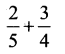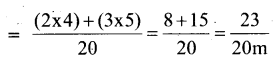Question 3.
Naina was given 1$$\frac{2}{5}$$ piece of cake and Najma was given 1$$\frac{1}{3}$$ piece of cake. Find the total amount of cake was given to both of them.
Solution:
Fraction Naina got = $$1 \frac{1}{2}=\frac{3}{2}$$
Fraction Najma got = $$1 \frac{1}{3}=\frac{4}{3}$$
Total amount of cake to them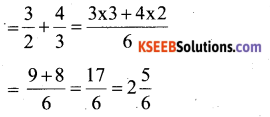Question 4.
Fill in the blanks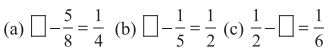Solution: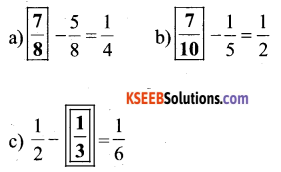Question 5.
Complete the addition – subtraction box.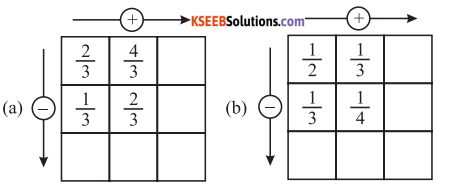Solution: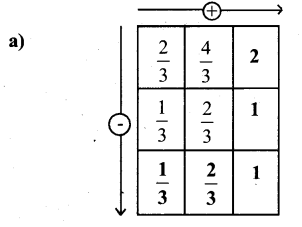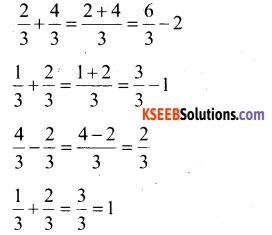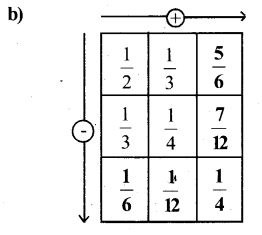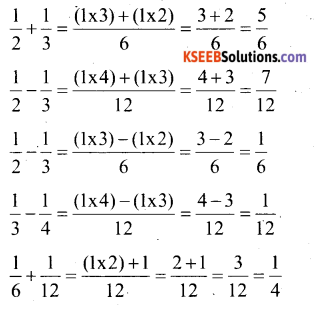Question 6.
A piece of wire $$\frac{7}{8}$$ metre long broke into two pieces. One piece was $$\frac{1}{4}$$ metre long. How long is the other piece ?
Solution:
Length of one piece = $$\frac{1}{4}$$ m
The length of the other piece of wire will be the difference of the length of the original wire
Hence, Length of the other piece of wire = $$\frac{7}{8}-\frac{1}{4}=\frac{7-(1 \times 2)}{8}-\frac{7-2}{8}=\frac{5}{8} \mathrm{m}$$

Question 7.
Nandini’s house is $$\frac{9}{10}$$ km from her school. She walked some distance and then took a bus for $$\frac{1}{2}$$ km to reach the school. How far did she walk?
Solution:
Distance walked by Nandhini = Total distance – Distance for Which she took the bus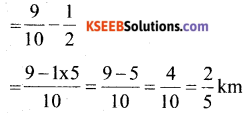Question 8.
Asha and Samuel have book shelves of the same size partly filled with books. Asha’s shelf is $$\frac{5}{6}$$ th full and samuel’s shelf is $$\frac{2}{5}$$ th full. Whose bookshelf is more full? By what fraction?
Solution: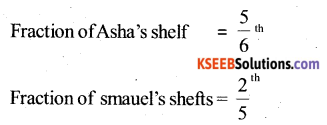Converting these into like fractions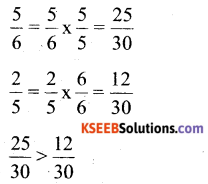Clearly, Asha’s bookshelf is more full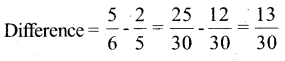Question 9.
Jaidev takes 2 $$\frac{1}{5}$$ minutes to walk across the school ground. Rahul takes $$\frac{7}{4}$$ to do the same. Who takes less time and by What fraction?
Solution:
Time taken by Jaidev = 2$$\frac{1}{5}$$ minutes = $$\frac{11}{5}$$ min
Time taken by Rahul = $$\frac{7}{4}$$ min
Converting these into like fractions.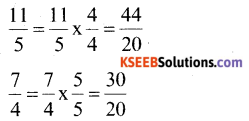As 44 > 35
Hence, Rahul takes lesser time